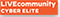# Palo decrypt error unsupported

cancel
Showing results for
Did you mean:
Announcements
We are conducting regularly scheduled maintenance over the weekend, which could cause some downtime on LIVEcommunity. We apologize for any inconvenience.

## Palo decrypt error unsupported

L4 Transporter

Hi,

We are receiving decrypt error in our ssl inspection traffic

++++As Per the below logs Server is using an unsupported EC curve x25519. Correct the server configuration to use a curve that the firewall supports.++++

2021-03-17 06:59:01.789 +0100 Error: pan_tls_ec_curve_id_2nid(pan_ec_common.c:66): unsupported ec curve_id 29<<<<<<<<<<<<<<<<<<<<<<<<<<<<<<<<<<<<<<<<<<<<<<<<
2021-03-17 06:59:01.789 +0100 Error: pan_ecdh_parse_server_key_exchange_msg(pan_ecdh.c:436): unsupported curve_na
me 29.<<<<<<<<<<<<<<<<<<<<<<<<<<<<<<<<<<<<<<<<<<<<<<<<<<<<<<<<<<<<<<<<<<<<<<<<<<<<<<<<<<<<<<<<<<<<<<<<<<<<<<<<<<<<<<<<<<<<<<<<<<<<<<<<<<<
2021-03-17 06:59:01.789 +0100 Error: pan_ssl_keyxchg_parse_server_key_exchange_msg(pan_ssl_keyxchg.c:166): parse_
server_key_exchange_msg(ecdhe) failed<<<<<<<<<<<<<<<<<<<<<<<<<<<<<<<<<<<<<<<<<<<<<<<<<<<<<<<<<<<<<<<<<<<<<<<<<<<<<<<<<<<<<<<<<<<<<<<<<<<

PAN-OS 8.1 Decryption Cipher Suites
>>https://docs.paloaltonetworks.com/compatibility-matrix/supported-cipher-suites/cipher-suites-support...

-------

I still don't understand why the PA generates a "decryption error" instead of letting the connection go through. During testing there were some cases where the client supported TLS 1.3. As the servers also support it and the AP does not, the connection just worked. In this case, the "conflicting" information does not arrive in the "Server hello" message but just after the "key exchange" message, but since the Server hello message is not transmitted to the client, you should undo the session proxy and forward the original message from the server to the client. Anyway, I guess it can't be done for some reason.

any wway to solve it?

2 REPLIES 2Cyber Elite

Hi @BigPalo

What PAN-OS version do you use? Could you tell us the website where you see this decryption error?Cyber Elite

If your firewall is 8.1 as you have given an article for it then you will not be able to decrypt TLS1.3. Read this:

https://docs.paloaltonetworks.com/pan-os/10-0/pan-os-new-features/decryption-features/ssl-decryption...

Also in version 10 there is a new log for SSL decryption issues: